Download Presentation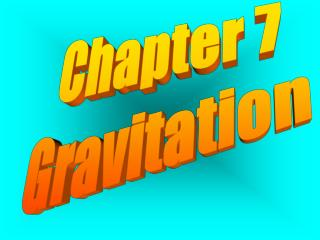Chapter 7 Gravitation

# Chapter 7 Gravitation - PowerPoint PPT Presentation

Chapter 7 Gravitation. 7.1 Planetary Motion. Planets move through the sky with More complicated paths than Stars do. . We begin with Kepler’s Laws in Order to find out how. Kepler’s Laws of planetary motion Are stated as… . The paths of planets are ellipses, With the sun at one focus.I am the owner, or an agent authorized to act on behalf of the owner, of the copyrighted work described.
Download Presentation## Chapter 7 Gravitation

An Image/Link below is provided (as is) to download presentation

Download Policy: Content on the Website is provided to you AS IS for your information and personal use and may not be sold / licensed / shared on other websites without getting consent from its author.While downloading, if for some reason you are not able to download a presentation, the publisher may have deleted the file from their server.

- - - - - - - - - - - - - - - - - - - - - - - - - - E N D - - - - - - - - - - - - - - - - - - - - - - - - - -
Presentation Transcript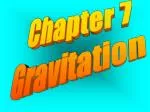Chapter 7

Gravitation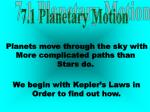7.1 Planetary Motion

Planets move through the sky with

More complicated paths than

Stars do.

We begin with Kepler’s Laws in

Order to find out how.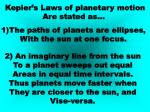Kepler’s Laws of planetary motion

Are stated as…

• The paths of planets are ellipses,
• With the sun at one focus.

2) An imaginary line from the sun

To a planet sweeps out equal

Areas in equal time intervals.

Thus planets move faster when

They are closer to the sun, and

Vise-versa.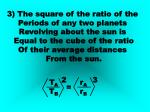3) The square of the ratio of the

Periods of any two planets

Revolving about the sun is

Equal to the cube of the ratio

Of their average distances

From the sun.

2

3

TA

TB

rA

rB

=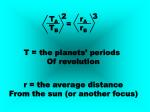2

3

TA

TB

rA

rB

=

T = the planets’ periods

Of revolution

r = the average distance

From the sun (or another focus)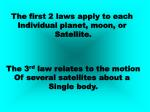The first 2 laws apply to each

Individual planet, moon, or

Satellite.

The 3rd law relates to the motion

Of several satellites about a

Single body.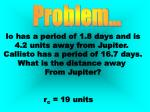Problem...

Io has a period of 1.8 days and is

4.2 units away from Jupiter.

Callisto has a period of 16.7 days.

What is the distance away

From Jupiter?

rc = 19 units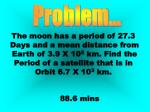Problem...

The moon has a period of 27.3

Days and a mean distance from

Earth of 3.9 X 105 km. Find the

Period of a satellite that is in

Orbit 6.7 X 103 km.

88.6 mins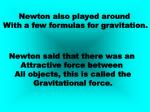Newton also played around

With a few formulas for gravitation.

Newton said that there was an

Attractive force between

All objects, this is called the

Gravitational force.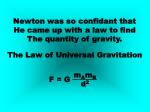Newton was so confidant that

He came up with a law to find

The quantity of gravity.

The Law of Universal Gravitation

mAmB

d2

F = GmAmB

d2

F = G

m = mass

d = distance between

The center of the objects

G = 6.67 X 10-11 Nm2 / kg2Without showing all the math,

The force equals something else.

mp4π2r

T2

msmp

d2

F =

= G

And rearranged again…

4π2

Gms

T2 =

r3Problem...

Jeff (75 kg) and Racheal (60 kg)

sit about 4 Meters away

from each other.

Racheal looks up and feels an

Attraction. Find the gravitational

Force between them.

F = 1.87 X 10-8 N7.2 Using the Law of

Universal Gravitation

An object that is falling at the

Same rate at which the earth is

Curved is said to be in orbit.Use Newton’s thought experiment

On page 179 to see how.

For an object to be in orbit,

air resistance Must be taken away,

which Means that it must

be at least 150 km

above the earth.We can continuously derive

New formulas to find out things.

GmE

r

v =

r3

GmE

T = 2π

These formulas only

Work for the earth.Problem...

A satellite orbits Earth 225 km

Above its surface. What is its

Speed in orbit and its period?

v = 7760 m/s T = 1.5 hrAs you move farther from Earth’s

Center, the acceleration due

To gravity changes.

2

rE

d

a = gThere are 2 kinds of mass…

Inertial mass

And

Gravitational massInertial mass is a measure of an

Object’s resistance to an type

Of force.

Fnet

a

minertial =The gravitational mass is the size

Of the gravitational force

Between the objects.

r2Fgrav

Gm

mgrav =Einstein proposed that gravity

Is not a force, but an effect

Of space itself.

According to Einstein, mass

Changes space itself,

Making it curved.Bodies are thus accelerated

Because they follow this

Curved space toward the center.

This is Einstein’s Special

Theory of Relativity.